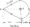# Field Theory December 2010

Note: Answer any FIVE full questions.

1 a. State and explain Coulomb’s law. Clearly indicate the unit of quantities used in the force equation.

b.   State and prove the Gauss theorem.

c.   Find E at P(l, 2, 3) due to Q3 – 5jnCat (-1, -2, -3) and Q2 = 1 Oj^C at (3, 5, -1).

2 a. Obtain an equation for the electric scalar potential.

b.  Show that the electric field intensity is a negative of the gradient of the electric scalar potential.

c.  Check whether the following potential equations are satisfying Laplace’s equation or not

i) V = 20x2yz +10xy2z2

ii) V = 15x2 +10y2 ~25z2

d.  Define : i) Electric field intensity ii) Displacement flux density.

3 a.  Derive Poisson’s and Laplace’s equations.

b.  Derive equations of energy stored and energy density in an electrostatic field.

c.   Discuss the boundary conditions between two different dielectrics.

4 a. Obtain the differential form of Ampere’s work law, in a steady magnetic field.

b.  By using Biot-Savart’s law, obtain the equations of magnetic field intensity and magnetic flux density at any point on the axis of a coil.

c.   Find the magnetic field intensity and the magnetic flux density at P, as shown in the figure Q4 (c).5 a.  Prove that V x E = —.

b.  For a time varying field, having a capacitor, show that, the conduction current is equal to the displacement current.

c.   Find the depth of penetration, when a 20 MHz signal is propagating the free space and penetrating into a conductor of conductivity cr = 5 x 107 O/m.

d- Write Maxwell’s equation in,

ii)  Time varying field.

6 a. State and prove the pointing vector theorem.

b.  Derive the wave equation of E & H.

c.   The electric field intensity at 10 km in free space from a radio station was found to be 2.2 mv/m. Calculate : i) The power density ii) The total power radiated from the station. Assume the radiation to be spherically symmetric.

7 a.  Show that the uniform plane wave is transverse in nature.

b.  Discuss the wave propagation in a good dielectric (absorption medium).

c.   A 10 MHz signal with Ex ~ 100 mV/m is propagating in a nature of medium with er~ 1.5 and \xT = 3.5. Find, i) Velocity ii) Phase constant iii) Wavelength iv) Intrinsic impedance and v)Hz.

8 a. Derive an equation for the force acting as a current element.

b.  Derive an equation for the torque as a closed circuit.

c.   Explain : i) Scalar magnetic potential ii) Vector magnetic potential.

d.  Derive an equation for the inductance of a solenoid.